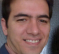## Goertzel algorithmStarted by 6 years ago4 replieslatest reply 6 years ago393 views

Hi,

For Goertzel algorithm, does it require N+1 or N samples to generate the bin corresponding to N-point DFT?

In Rick's book I see the following statement "For the Goertzel algorithm we retain only every Nth, or (N+1)th,". I am not sure why it "N+1 or N". Could someone please clarify?

Thanks,

Krishna

[ - ]Hi,

The Goertzel difference equations are

w(n)=2cos(2\pi m/N) w(n-1) - w(n-2) + x(n)

y(n)=w(n) - e^(-j2 \pi m/N) * w(n-1)

To find X(m), we need to calculate y(N), so we should find w(N) and w(N-1). Then the first equation should be evaluated N+1 times (assuming that the samples of x(n) start from index n=0 to n=N-1). However, if we are looking for the abs(X(m))^2, we can obtain the following equation from y(n) given above

abs(X(m))^2 = w(N-1)^2 + w(N-2)^2 - w(N-1)*w(N-2)*2*cos(2* \pi *m/N)

this is equation (13-83) of the book. Now, using this equation, we need to evaluate the w(n) equation by N times.

Figure 13-44 shows an example with N=64, for X(15) and abs(X(15)) the first stage should be evaluated by 65 and 64 times, respectively.

Please verify and correct me if I am making any mistake.

[ - ]Thanks Tavassoli.

I am just wondering if DFT/FFT and Goertzel evaluates the same bin, why should Goertzel depend on additional sample?

[ - ]DFT/FFT and Goertzel are different methods to calculate the same thing and Goertzel does not need any additional input sample. When we evaluate w(n) equation N+1 times, we are considering x(N)=0 as explained on page 530 of Rick's book and we are not using any new sample. (To see why x(N) should be set to zero, review the proof of the algorithm. To derive a convolution from DFT equation we have to assume x(n)=0 for n<0 and n>=N).

-Mahdi

[ - ]I couldn't tell you exactly how without wrapping myself in math for a while, but if you mind your p's and q's you should only need $$N$$ points.  Get the math right and the Goertzel has the exact same effect as doing a one-bin FFT (you can even extract both inphase and quadrature parts -- if you get the math right).